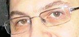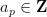[top] [TitleIndex] [WordIndex]

# Student Research

See my research blog for project ideas.

## Current Students (who I mentor)

All students listed below are at University of Washington, unless otherwise noted.

• Alyson Deines (Ph.D. student):
• Kolyvagin's conjecture over totally real fields
• Joanna Gaski (Masters thesis student)

## Recent Students (who I played a nontrivial role mentoring)

• Robert Bradshaw (Ph.D. student, expected Ph.D. 2010):
• Thesis: Computing L-functions
• Rigorous computation with complex-analytic L-functions (building on work of Dokchitser).
• Explicit investigations into p-adic analogues of the Birch and Swinnerton-Dyer conjecture for jacobians of genus 2 curves
• Coleman integration
• p-adic heights and Monsky-Washnitzer cohomology
• Symbolic algebra (automatic coercion)
• Compilers (http://www.cython.org)

• Asymptotically fast exact linear algebra
• Robert Miller (Ph.D. student, expected Ph.D. 2010):
• Thesis: Computational Verification of the Birch and Swinnerton-Dyer Conjecture
• Construction of rational points on high-rank elliptic curves using congruences and Galois cohomology
• Computation of canonical labelings of graphs
• Tom Boothby (Masters student):
• Computing L-functions to high precision
• Multiplication of matrices over GF(3^k)
• Fast polynomial evaluation
• Tables of modular forms
• Jennifer Balakrishnan (Ph.D. student at MIT):
• Explicit computation of Kolyvagin classes
• Senior Thesis on the relationships between the Convergence Sato-Tate Conjecture and the Generalized Riemann Hypothesis. In particular, a focus on the implication of the latter conjecture from the former. Examining different formulations of Sato-Tate.
• Computations related to the Sato-Tate conjecture
• Weierstrass equations for elliptic curves [mostly done]
• Very high-precision evaluation of L-series of elliptic curves of high rank
• Dimitar Jetchev (Ph.D., Berkeley)
• Ph.D. thesis improving on Kolyvagin's Sha bounds
• Explicit computation of Heegner points and Kolyvagin classes
•for higher degree newforms